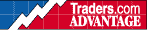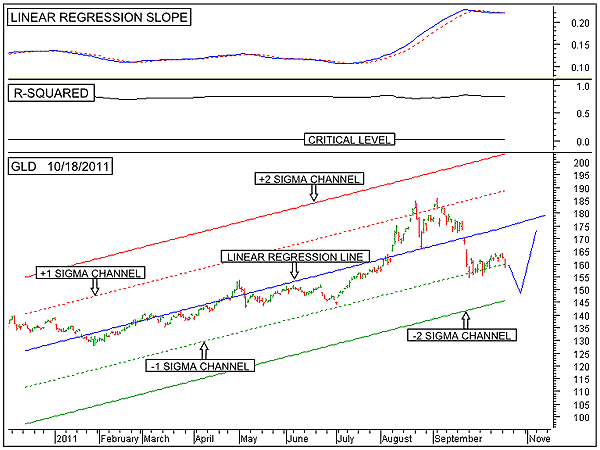HOT TOPICS LIST

INDICATORS LIST

LIST OF TOPICS

# Gold Remains In Long-Term Uptrend

10/19/11 09:01:40 AM
by Alan R. Northam

This long-term statistical analysis shows that gold remains in a long-term uptrend.

Security:   GLD
Position:   N/A

 Within the discipline of statistical analysis, the purpose of analyzing data is to determine if the data shows a trend and whether a prediction about the future can be made. In making a prediction about the future, statisticians calculate the coefficient of determination before doing anything else. The coefficient of determination is then squared to eliminate negative values and make the indicator more sensitive. The R-squared indicator in the middle panel of Figure 1 is such an indicator. In order to make reliable predictions about the future, the R-squared indicator needs to be above its critical value. If the data shows that the R-squared value is above it, the data is said to be statistically significant and a linear regression line can then be drawn. The linear regression line is then used to make a predication about the future. It is a "no-no" in the field of statistics to use a linear regression line to make a prediction about the future when the R-squared value is below its critical level. The lower panel of Figure 1 shows the long-term statistical analysis of the SPDR Gold Shares ETF (GLD). This figure shows the 200-day linear regression trendline (solid blue line), the upper one sigma channel line (dotted red line), the upper two sigma channel line (solid red line), the lower one sigma channel line (dotted green line), and the lower two sigma channel line (solid green line). Besides their statistical significance, the red channel lines act as resistance and the green channel lines support.FIGURE 1: GLD, DAILY. This chart shows the daily price chart of the SPDR Gold Shares ETF in the lower panel along with its upsloping 200-day linear regression trendline and its associated upper and lower channel lines. The top panel shows the linear regression slope indicator followed by the R-squared indicator. This chart shows that GLD remains in a long-term uptrend. Graphic provided by: MetaStock. Figure 1 shows that over the last 200 daily trading sessions, GLD has, for the most part, traded between its upper and lower one sigma channel lines. The significance of these lines is that they represent the boundaries in which price trades 68% of the time. This statistic then means that 32% of the time, price trades outside these boundaries. Since price has been trading within the boundaries of the upper and lower one sigma channel lines over the last 200 daily sessions, logic tells us that at some point in the future, price is either going to break out above the upper one sigma channel line or break down below the lower one sigma channel line. Looking at the R-squared indicator in the middle panel of Figure 1 shows a reading of 0.83, a strong reading well above its critical level. Note that this indicator moves between zero and one. A strong reading like this is an indication that we can make a reliable predication about the future price of GLD. In looking back at the lower panel of Figure 1, we see that the linear regression line (solid blue line) continues to move higher as it is projected into the future. This line represents a reasonable price target for GLD. As a result, over the long term, we should expect price to move up toward this linear regression line. Although our prediction shows that price should move upward, there is something else going on that we need to be aware of. Looking at the linear regression slope indicator in the top panel of Figure 1, note that from mid-September onward, this indicator has started to move downward. This indicates that the slope of the linear regression line in the bottom panel is starting to become more shallow. Therefore, as price is predicted to move up and toward the linear regression line, the linear regression line itself is starting to move downward and toward price as its slope becomes more and more shallow. At some point, price and the linear regression line will converge upon each other.Predictions about the future is just that; predictions. They are not guarantees. Predictions are a guide for the trader to use in making trading decisions. This statistical analysis shows that over the long term, price is predicted to rally and toward the linear regression line, while at the same time, the linear regression line is becoming more shallow and moving toward price converging upon each other. This analysis also stated that at some point in the future, price will break down below the one sigma channel line. My shorter-term statistical analysis (not shown) predicts that price will move downward and break below the lower one sigma 200-day channel line (green dotted line) as it completes its downward move before starting to rally back toward the 200-day linear regression line.

Alan R. Northam

Alan Northam lives in the Dallas, Texas area and as an electronic engineer gave him an analytical mind from which he has developed a thorough knowledge of stock market technical analysis. His abilities to analyze the future direction of the stock market has allowed him to successfully trade of his own portfolio over the last 30 years. Mr. Northam is now retired and trading the stock market full time. You can reach him at inquiry@tradersclassroom.com or by visiting his website at http://www.tradersclassroom.com. You can also follow him on Twitter @TradersClassrm.

 Comments or Questions? Article Usefulness 5 (most useful) 4 3 2 1 (least useful)